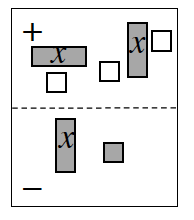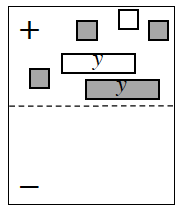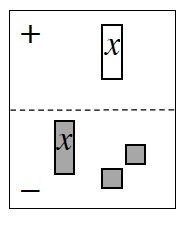### Home > CAAC > Chapter 2 > Lesson 2.1.4 > Problem2-44

2-44.

Write and simplify the algebraic expression shown in each Expression Mat below.1.$\left(2x − 3\right) − \left( ? \right)$

1.Which tile pairs make zeroes?

1.$−x −\left(x + 2\right) = −2x − 2$